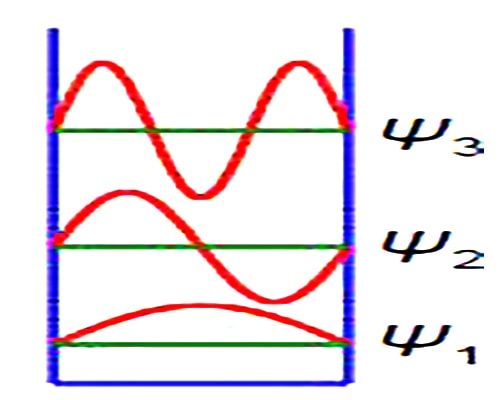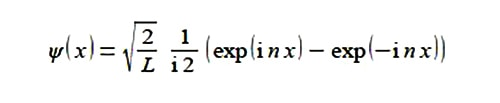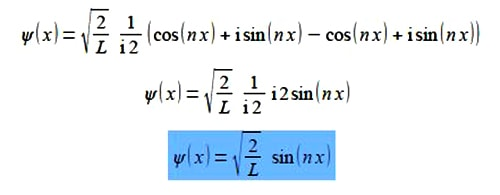# Particle in A One-Dimensional Box

Quantum effects are dominant in many areas of Chemistry and Biology. A simple system that shows how quantum effects arise is the particle in a box. The particle may be an electron, atom, ion or molecule dependent upon the system being studied. The first three wavefunctions for n =1, 2, and 3 are shown in Figure which has a vertical axis not to scale. The wavefuction increases and decreases with the distance along the One-Dimensional Box and thus is best modelled using complex numbers.

The wavefimctions ψ for the particle in a one dimensional box are given by the following complex-functionWhere L is the length of the box, xis the position of the particle within the One-Dimensional Box and n is a constant called the transnational quantum number n = 1,2, 3, 4, … Simplify this expression to obtain a real valued function.SolutionSubstituting from Euler’s formulae written in terms of (inx) we have,This is a real wavefunction.